## 5.3.3 Incremental Methods

This section focuses on a particular approach called incremental distance computation, which assumes that between successive calls to the collision detection algorithm, the bodies move only a small amount. Under this assumption the algorithm achieves almost constant time'' performance for the case of convex polyhedral bodies [636,702]. Nonconvex bodies can be decomposed into convex components.

These collision detection algorithms seem to offer wonderful performance, but this comes at a price. The models must be coherent, which means that all of the primitives must fit together nicely. For example, if a 2D model uses line segments, all of the line segments must fit together perfectly to form polygons. There can be no isolated segments or chains of segments. In three dimensions, polyhedral models are required to have all faces come together perfectly to form the boundaries of 3D shapes. The model cannot be an arbitrary collection of 3D triangles.

The method will be explained for the case of 2D convex polygons, which are interpreted as convex subsets of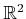. Voronoi regions for a convex polygon will be defined in terms of features. The feature set is the set of all vertices and edges of a convex polygon. Thus, a polygon withedges has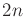features. Any point outside of the polygon has a closest feature in terms of Euclidean distance. For a given feature,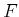, the set of all points infrom whichis the closest feature is called the Voronoi region ofand is denoted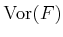. Figure 5.11 shows all ten Voronoi regions for a pentagon. Each feature is considered as a point set in the discussion below.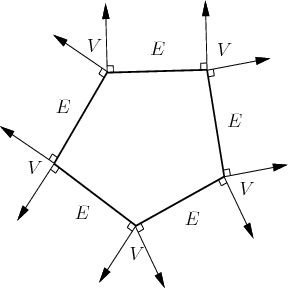For any two convex polygons that do not intersect, the closest point is determined by a pair of points, one on each polygon (the points are unique, except in the case of parallel edges). Consider the feature for each point in the closest pair. There are only three possible combinations:

• Vertex-Vertex Each point of the closest pair is a vertex of a polygon.
• Edge-Vertex One point of the closest pair lies on an edge, and the other lies on a vertex.
• Edge-Edge Each point of the closest pair lies on an edge. In this case, the edges must be parallel.

Let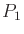and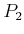be two convex polygons, and let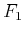and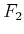represent any feature pair, one from each polygon. Let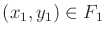and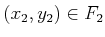denote the closest pair of points, among all pairs of points inand, respectively. The following condition implies that the distance between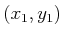and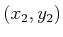is the distance betweenand: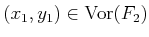and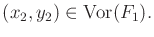(5.29)

If (5.29) is satisfied for a given feature pair, then the distance betweenandequals the distance betweenand. This implies that the distance betweenandcan be determined in constant time. The assumption thatmoves only a small amount relative tois made to increase the likelihood that the closest feature pair remains the same. This is why the phrase almost constant time'' is used to describe the performance of the algorithm. Of course, it is possible that the closest feature pair will change. In this case, neighboring features are tested using the condition above until the new closest pair of features is found. In this worst case, this search could be costly, but this violates the assumption that the bodies do not move far between successive collision detection calls.

The 2D ideas extend to 3D convex polyhedra [247,636,702]. The primary difference is that three kinds of features are considered: faces, edges, and vertices. The cases become more complicated, but the idea is the same. Once again, the condition regarding mutual Voronoi regions holds, and the resulting incremental collision detection algorithm has almost constant time'' performance.

Steven M LaValle 2012-04-20